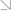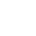全国热线：400-963-0708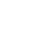网站地图 Sign in Sign up

# CFA二级考纲中的Quantitative Method科目变化是怎样的？CFA二级考纲中的Quantitative Method科目变化是怎样的，2022CFA考纲已经出来，报考2022CFA二级考试的考生要选弄清楚CFA二级考纲的变化，那是怎样的呢？和小编一起看看吧！a) distinguish between the dependent and independent variables in a linear regression;

b） explain the assumptions underlying linear regression and interpret regression coefficients;

c） calculate and interpret the standard error of estimate, the coefficient of determination,and a confidence interval for a regression coefficient;

d) formulate a null and alternative hypothesis about a population value of a regression coefficient and determine the appropriate test statistic and whether the null hypothesis is rejected at a given level of significance;

e） calculate the predicted value for the dependent variable, given an estimated regression model and a value for the independent variable;

f) calculate and interpret a confidence interval for the predicted value of the dependent variable;

g) describe the use of analysis of variance (ANOVA) in regression analysis, interpret ANOVA results, and calculate and interpret the F-statistic;

h) describe limitations of regression analysis.

a) describe a simple linear regression model and the roles of the dependent and independent variables in the model;

b) describe the least squares criterion, how it is used to estimate regression coefficients, and their interpretation;

c) explain the assumptions underlying the simple linear regression model, and describe how residuals and residual plots indicate if these assumptions may have been violated;

d) calculate and interpret the coefficient of determination and the F-statistic in a simple linear regression;

e) describe the use of analysis of variance (ANOVA) in regression analysis, interpret ANOVA results, and calculate and interpret the standard error of estimate in a simple linear regression;

f) formulate a null and an alternative hypothesis about a population value of a regression coefficient, and determine whether the null hypothesis is rejected at a given level of significance;

g） calculate and interpret the predicted value for the dependent variable, and a prediction interval for it, given an estimated linear regression model and a value for the independent variable;

h） describe different functional forms of simple linear regressions.

e) calculate and interpret 1) a confidence interval for the population value of a regression coefficient and 2) a predicted value for the dependent variable, given an estimated regression model and assumed values for the independent variables;

CFA备考怎么能少了CFA备考资料呢？小编为各位考生准备了CFA备考资料，有需要可以点击下方链接获取！j )formulate a multiple regression equation by using dummy variables to represent qualitative factors and interpret the coefficients and regression results

CFA考试知识点的内容就这么多，学员如果对知识点还有更多的疑问，可以咨询CFA学习交流群(扫码免费加群)

•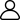1、新手入门
•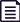2、学习资料
•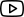3、免费课程
•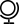4、考试动态
•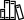5、备考干货
•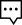6、答疑冲刺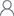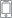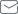2021年8月13日CFA一级考试 还有

81
18小时 45分钟 36

## 推荐课程